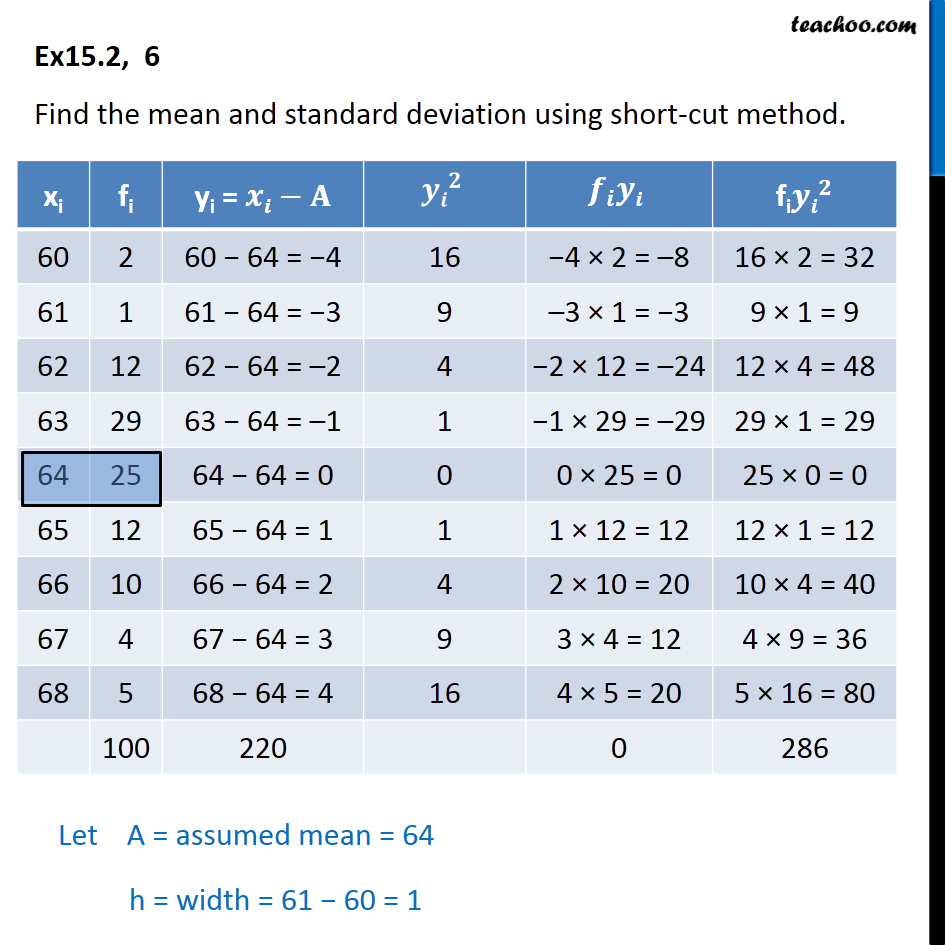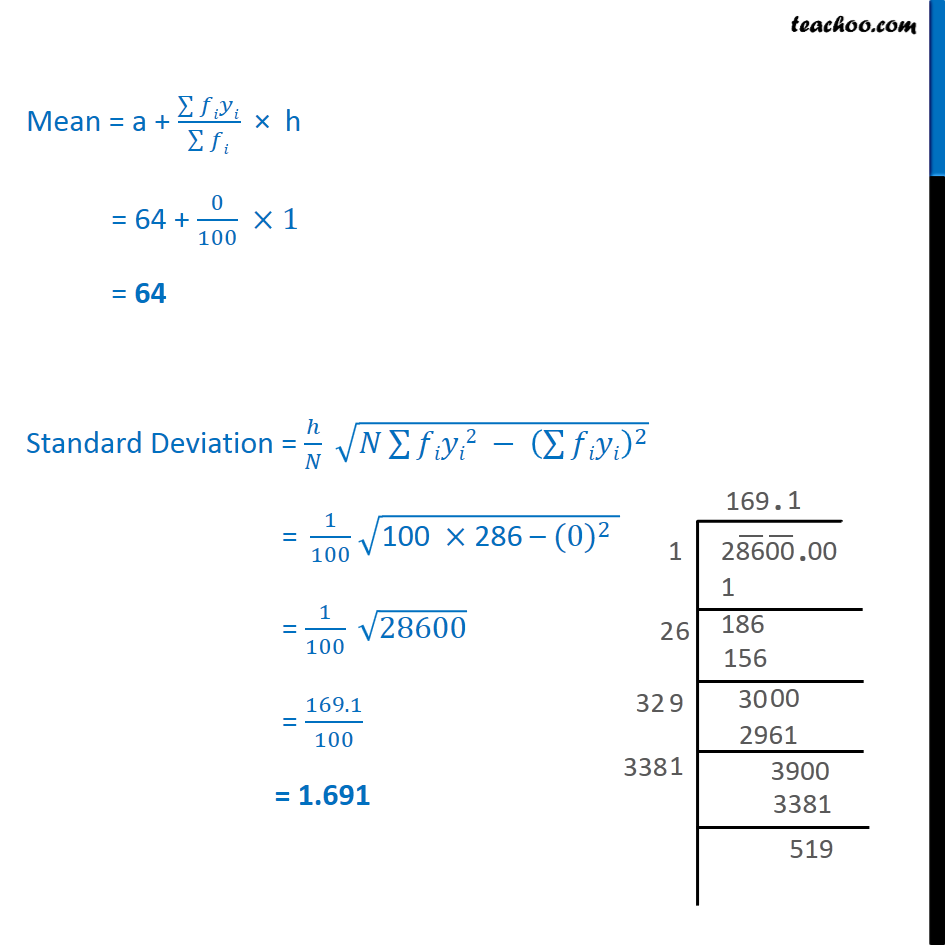Ex 15.2

Chapter 15 Class 11 Statistics
Serial order wiseMaths Crash Course - Live lectures + all videos + Real time Doubt solving!

### Transcript

Ex 15.2, 6 Find the mean and standard deviation using short-cut method. Mean = a + (∑▒𝑓𝑖𝑦𝑖)/(∑▒𝑓𝑖) × h = 64 + 0/100 ×1 = 64 Standard Deviation = ℎ/𝑁 √(𝑁∑▒𝑓𝑖𝑦𝑖2 − (∑▒𝑓𝑖𝑦𝑖)^2 ) "= " 1/100 √("100" ×"286 – " 〖(0)〗^2 ) = 1/100 √28600 = 169.1/100 = 1.691